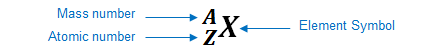# Problem: Identify the element represented by each of the following symbols and give the number of protons and neutrons in each.Identify the element 3374 X.

###### FREE Expert Solution

We’re being asked to identify the element, number of protons and number of neutrons in 3374X.

An element can be represented as:You can determine the identity of the element using its atomic number:

Atomic number (Z):

represents the identity of the element
reveals the number of protons
in a neutral element:

# of protons = # of electrons

Mass number (A)

reveals the number of protons and neutrons

mass number = # of protons + # of neutrons

Given: 3374X

87% (357 ratings)###### Problem Details

Identify the element represented by each of the following symbols and give the number of protons and neutrons in each.

Identify the element 3374 X.